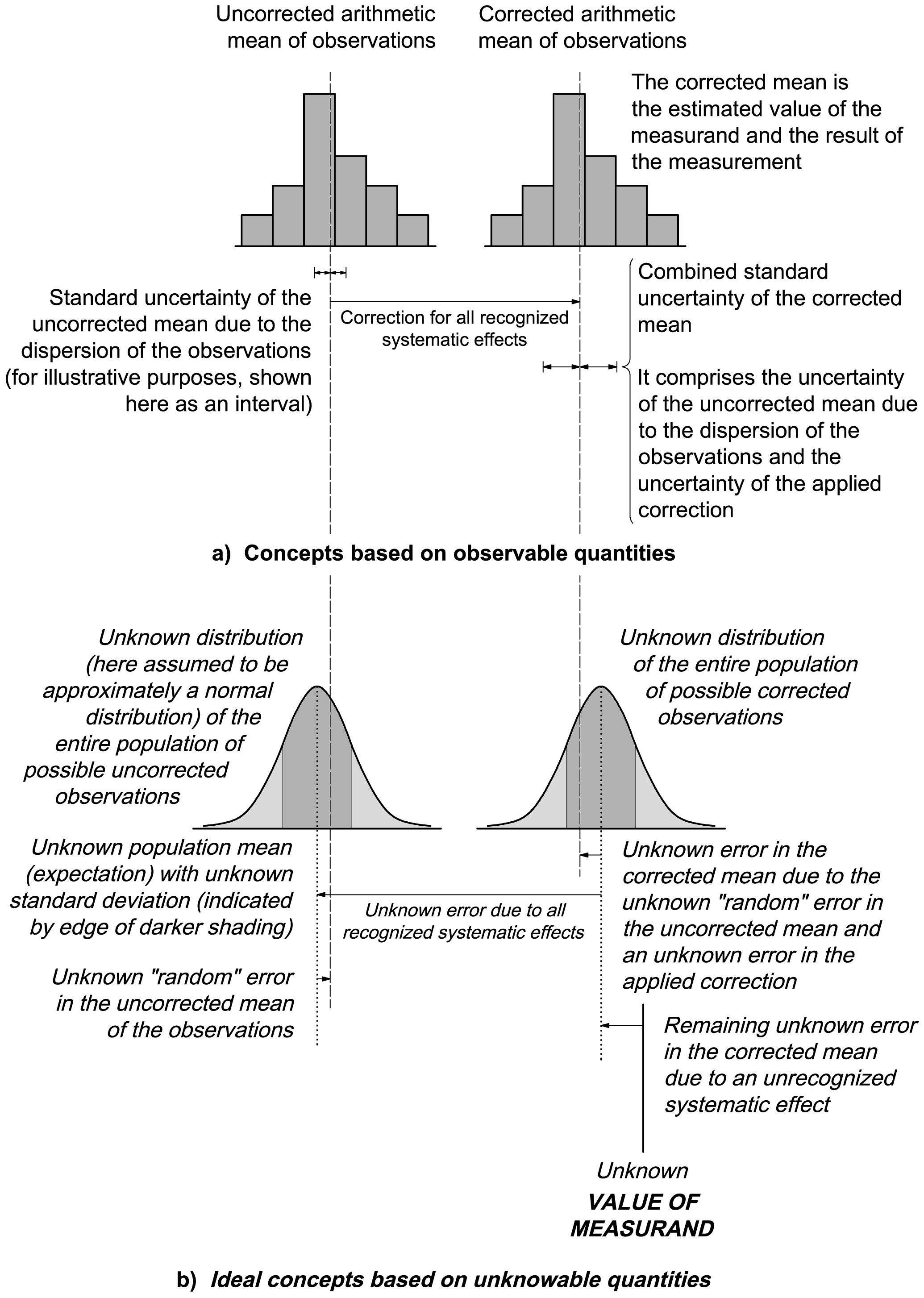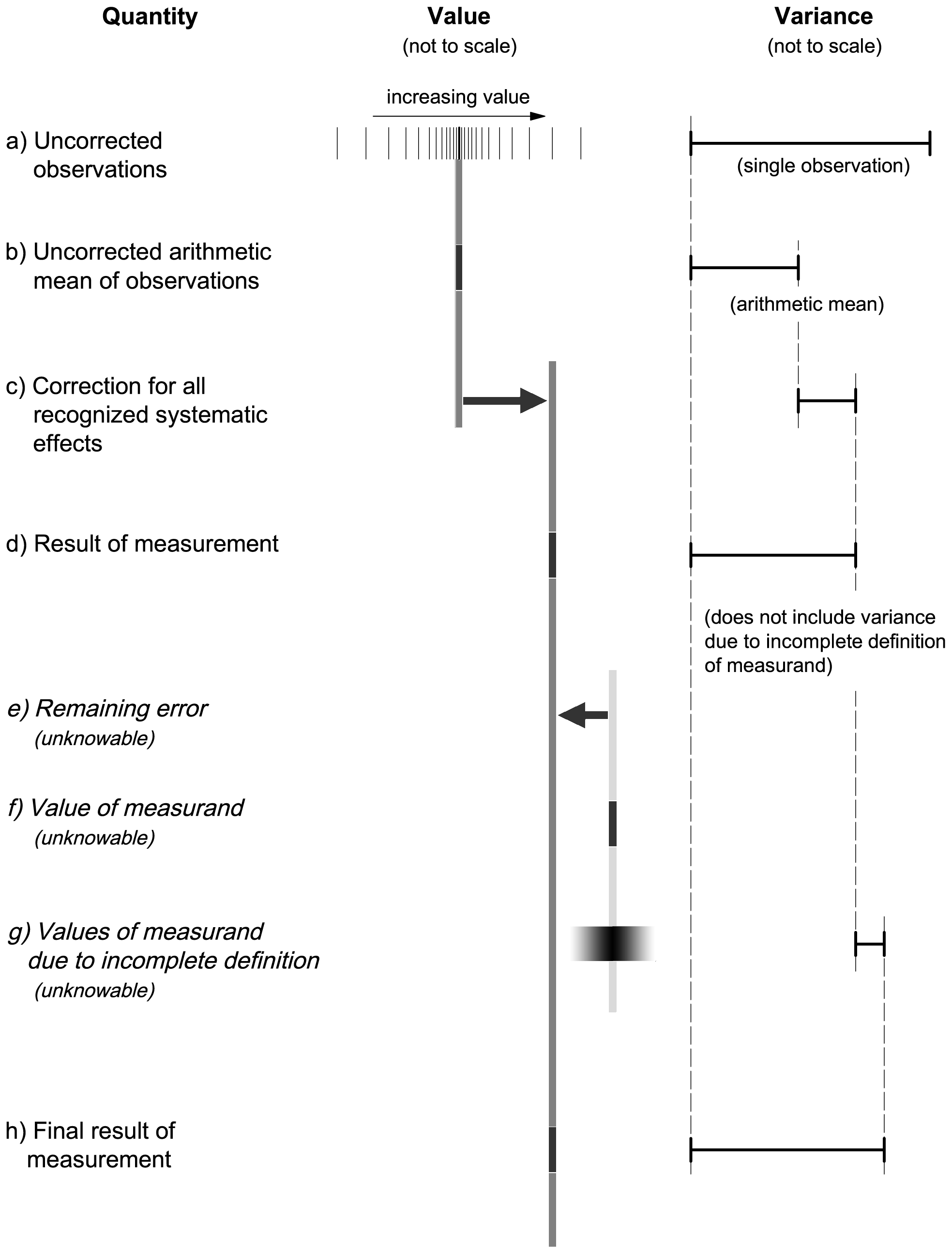Annex D “True” value, error, and uncertainty

The term true value (B.2.3) has traditionally been used in publications on uncertainty but not in this Guide for the reasons presented in this annex. Because the terms “measurand”, “error”, and “uncertainty” are frequently misunderstood, this annex also provides additional discussion of the ideas underlying them to supplement the discussion given in Clause 3. Two figures are presented to illustrate why the concept of uncertainty adopted in this Guide is based on the measurement result and its evaluated uncertainty rather than on the unknowable quantities “true” value and error.

D.1   The measurand

D.1.1   The first step in making a measurement is to specify the measurand — the quantity to be measured; the measurand cannot be specified by a value but only by a description of a quantity. However, in principle, a measurand cannot be completely described without an infinite amount of information. Thus, to the extent that it leaves room for interpretation, incomplete definition of the measurand introduces into the uncertainty of the result of a measurement a component of uncertainty that may or may not be significant relative to the accuracy required of the measurement.

D.1.2   Commonly, the definition of a measurand specifies certain physical states and conditions.

EXAMPLE   The velocity of sound in dry air of composition (mole fraction) N2 = 0,7808, O2 = 0,2095, Ar = 0,009 35, and CO2 = 0,000 35 at the temperature T = 273,15 K and pressure p = 101 325 Pa.

D.2   The realized quantity

D.2.1   Ideally, the quantity realized for measurement would be fully consistent with the definition of the measurand. Often, however, such a quantity cannot be realized and the measurement is performed on a quantity that is an approximation of the measurand.

D.3   The “true” value and the corrected value

D.3.1   The result of the measurement of the realized quantity is corrected for the difference between that quantity and the measurand in order to predict what the measurement result would have been if the realized quantity had in fact fully satisfied the definition of the measurand. The result of the measurement of the realized quantity is also corrected for all other recognized significant systematic effects. Although the final corrected result is sometimes viewed as the best estimate of the “true” value of the measurand, in reality the result is simply the best estimate of the value of the quantity intended to be measured.

D.3.2   As an example, suppose that the measurand is the thickness of a given sheet of material at a specified temperature. The specimen is brought to a temperature near the specified temperature and its thickness at a particular place is measured with a micrometer. The thickness of the material at that place and temperature, under the pressure applied by the micrometer, is the realized quantity.

D.3.3   The temperature of the material at the time of the measurement and the applied pressure are determined. The uncorrected result of the measurement of the realized quantity is then corrected by taking into account the calibration curve of the micrometer, the departure of the temperature of the specimen from the specified temperature, and the slight compression of the specimen under the applied pressure.

D.3.4   The corrected result may be called the best estimate of the “true” value, “true” in the sense that it is the value of a quantity that is believed to satisfy fully the definition of the measurand; but had the micrometer been applied to a different part of the sheet of material, the realized quantity would have been different with a different “true” value. However, that “true” value would be consistent with the definition of the measurand because the latter did not specify that the thickness was to be determined at a particular place on the sheet. Thus in this case, because of an incomplete definition of the measurand, the “true” value has an uncertainty that can be evaluated from measurements made at different places on the sheet. At some level, every measurand has such an “intrinsic” uncertainty that can in principle be estimated in some way. This is the minimum uncertainty with which a measurand can be determined, and every measurement that achieves such an uncertainty may be viewed as the best possible measurement of the measurand. To obtain a value of the quantity in question having a smaller uncertainty requires that the measurand be more completely defined.

NOTE 1   In the example, the measurand's specification leaves many other matters in doubt that might conceivably affect the thickness: the barometric pressure, the humidity, the attitude of the sheet in the gravitational field, the way it is supported, etc.

NOTE 2   Although a measurand should be defined in sufficient detail that any uncertainty arising from its incomplete definition is negligible in comparison with the required accuracy of the measurement, it must be recognized that this may not always be practicable. The definition may, for example, be incomplete because it does not specify parameters that may have been assumed, unjustifiably, to have negligible effect; or it may imply conditions that can never be fully met and whose imperfect realization is difficult to take into account. For instance, in the example of D.1.2, the velocity of sound implies infinite plane waves of vanishingly small amplitude. To the extent that the measurement does not meet these conditions, diffraction and nonlinear effects need to be considered.

NOTE 3   Inadequate specification of the measurand can lead to discrepancies between the results of measurements of ostensibly the same quantity carried out in different laboratories.

D.3.5   The term “true value of a measurand” or of a quantity (often truncated to “true value”) is avoided in this Guide because the word “true” is viewed as redundant. “Measurand” (see B.2.9) means “particular quantity subject to measurement”, hence “value of a measurand” means “value of a particular quantity subject to measurement”. Since “particular quantity” is generally understood to mean a definite or specified quantity (see B.2.1, Note 1), the adjective “true” in “true value of a measurand” (or in “true value of a quantity”) is unnecessary — the “true” value of the measurand (or quantity) is simply the value of the measurand (or quantity). In addition, as indicated in the discussion above, a unique “true” value is only an idealized concept.

D.4   Error

A corrected measurement result is not the value of the measurand — that is, it is in error — because of imperfect measurement of the realized quantity due to random variations of the observations (random effects), inadequate determination of the corrections for systematic effects, and incomplete knowledge of certain physical phenomena (also systematic effects). Neither the value of the realized quantity nor the value of the measurand can ever be known exactly; all that can be known is their estimated values. In the example above, the measured thickness of the sheet may be in error, that is, may differ from the value of the measurand (the thickness of the sheet), because each of the following may combine to contribute an unknown error to the measurement result:

1. slight differences between the indications of the micrometer when it is repeatedly applied to the same realized quantity;
2. imperfect calibration of the micrometer;
3. imperfect measurement of the temperature and of the applied pressure;
4. incomplete knowledge of the effects of temperature, barometric pressure, and humidity on the specimen or the micrometer or both.

D.5   Uncertainty

D.5.1   Whereas the exact values of the contributions to the error of a result of a measurement are unknown and unknowable, the uncertainties associated with the random and systematic effects that give rise to the error can be evaluated. But, even if the evaluated uncertainties are small, there is still no guarantee that the error in the measurement result is small; for in the determination of a correction or in the assessment of incomplete knowledge, a systematic effect may have been overlooked because it is unrecognized. Thus the uncertainty of a result of a measurement is not necessarily an indication of the likelihood that the measurement result is near the value of the measurand; it is simply an estimate of the likelihood of nearness to the best value that is consistent with presently available knowledge.

D.5.2   Uncertainty of measurement is thus an expression of the fact that, for a given measurand and a given result of measurement of it, there is not one value but an infinite number of values dispersed about the result that are consistent with all of the observations and data and one's knowledge of the physical world, and that with varying degrees of credibility can be attributed to the measurand.

D.5.3   It is fortunate that in many practical measurement situations, much of the discussion of this annex does not apply. Examples are when the measurand is adequately well defined; when standards or instruments are calibrated using well‑known reference standards that are traceable to national standards; and when the uncertainties of the calibration corrections are insignificant compared to the uncertainties arising from random effects on the indications of instruments, or from a limited number of observations (see E.4.3). Nevertheless, incomplete knowledge of influence quantities and their effects can often contribute significantly to the uncertainty of the result of a measurement.

D.6   Graphical representation

D.6.1   Figure D.1 depicts some of the ideas discussed in Clause 3 of this Guide and in this annex. It illustrates why the focus of this Guide is uncertainty and not error. The exact error of a result of a measurement is, in general, unknown and unknowable. All one can do is estimate the values of input quantities, including corrections for recognized systematic effects, together with their standard uncertainties (estimated standard deviations), either from unknown probability distributions that are sampled by means of repeated observations, or from subjective or a priori distributions based on the pool of available information; and then calculate the measurement result from the estimated values of the input quantities and the combined standard uncertainty of that result from the standard uncertainties of those estimated values. Only if there is a sound basis for believing that all of this has been done properly, with no significant systematic effects having been overlooked, can one assume that the measurement result is a reliable estimate of the value of the measurand and that its combined standard uncertainty is a reliable measure of its possible error.

NOTE 1   In Figure D.1 a), the observations are shown as a histogram for illustrative purposes [see 4.4.3 and Figure 1 b)].

NOTE 2   The correction for an error is equal to the negative of the estimate of the error. Thus in Figure D.1, and in Figure D.2 as well, an arrow that illustrates the correction for an error is equal in length but points in the opposite direction to the arrow that would have illustrated the error itself, and vice versa. The text of the figure makes clear if a particular arrow illustrates a correction or an error.

D.6.2   Figure D.2 depicts some of the same ideas illustrated in Figure D.1 but in a different way. Moreover, it also depicts the idea that there can be many values of the measurand if the definition of the measurand is incomplete [entry g) of Figure D.2]. The uncertainty arising from this incompleteness of definition as measured by the variance is evaluated from measurements of multiple realizations of the measurand, using the same method, instruments, etc. (see D.3.4).

NOTE   In the column headed “Variance”, the variances are understood to be the variances u2i(y) defined in Equation (11a) in 5.1.3; hence they add linearly as shown.Figure D.1 — Graphical illustration of value, error, and uncertaintyFigure D.2 — Graphical illustration of values, error, and uncertainty